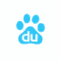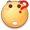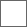• 5回复贴，共1

### 制作人物中的部分新手问题

[Statedef 0]
type = S
physics = S
sprpriority = 0

[State 0, 1]
type = ChangeAnim
triggerall = roundno = 1
trigger1 = Anim != 0 && Anim != 5
trigger2 = Anim = 5&& AnimTime = 0 ;Turn anim over
value = 0

[State 0, 5]
type = Explod
trigger1 = roundno = 2
trigger1 = anim = 2
trigger1 = time = 0
anim = 8
id = 2
sprpriority =2
postype = p1
pos = 61,-165
bindtime = -1
removetime = -1
supermove = 1
under = 0
ownpal = 1

[State 0, 12]
type = ChangeAnim
triggerall = roundno = 2
trigger1 = Anim != 2 && Anim != 5
trigger2 = Anim = 5 && AnimTime = 0 ;Turn anim over
value = 2

[State 0, 2]
type = VelSet
trigger1 = Time = 0
y = 0

[State 0, 3] ;Stop moving if low velocity or 4 ticks pass
type = VelSet
trigger1 = abs(vel x) < 2
trigger2 = Time = 4
x = 0

[State 0, 4]; PosSet + 0 Para evitar errores de posicion
type = PosSet
trigger1 = 1
y = 0

[State 0, PalFX]
type = PalFX
trigger1 = time = 0
time = 1
ignorehitpause = 1

[State 0, AfterImage]
type = AfterImage
trigger1 = time = 0
time = 1
ignorehitpause = 1

[State 0, 3] ;Stop moving if low velocity or 4 ticks pass
type = VelSet
trigger1 = abs(vel x) < Const(movement.stand.friction.threshold)
trigger2 = Time = 4
x = 0

[State 0, 5] ;Are you dead?
type = ChangeState
trigger1 = !alive
value = 5050

2020-01-20 01:21 广告
type = Explod里写了trigger1 = roundno = 2, 导致第二回合才能出特效anim =2？

type = Explod

type = ChangeAnim

#### 扫二维码下载贴吧客户端